## MP Board Class 9th Maths Solutions Chapter 14 Statistics Ex 14.4

Question 1.
The following number of goals were scored by a team in a series of 10 matches:
2, 3, 4, 5, 0, 1, 3, 3, 4, 3
Find the mean, median and mode of these scores.
Solution:
1.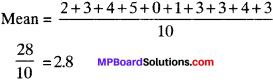2. Arranging the data in ascending order, we get
0, 1, 2, 3, 3, 3, 3, 4, 4, 5
n = 10 (even)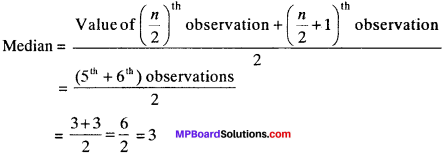3. As 3 is repeated maximum number of times i. e., 4 times.
∴ Mode = 3

Question 2.
In a mathematics test given to 15 students, the following marks (out of 100) are recorded:
41, 39, 48, 52, 46, 62, 54, 40, 96, 52, 98, 40, 42, 52, 60
Find the mean, median and mode of this data.
Solution:
1. Mean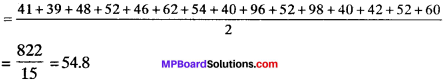2. Arranging the data in ascending order, we get
39, 40, 40, 41, 42, 46, 48, 52, 52, 52, 54, 60, 62, 96, 98
n = 15 (odd)
Median = ($$\frac{n+1}{2}$$)th obseravation
= ($$\frac{15+1}{2}$$)th observation = 8th obseravation
= 52.

3. As 52 is repeated maximum number of times i.e., 3 times
∴ Mode = 52Question 3.
The following observations have been arranged in ascending order. If the median of the data is 63, find the value of x.
29, 32, 48, 50, x, x + 2, 72, 78, 84, 95
Solution:
Median = 63 (given)
n = 10 (even)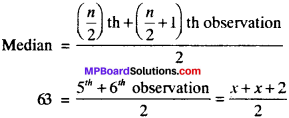2 x 63 = 2x + 2
⇒ 126 = 2x + 2
⇒ 126 – 2 = 2x
⇒ 7x = 124
x = 62

Question 4.
Find the mode of 14, 25, 14, 28, 18, 17, 18, 14, 23, 22, 14, 18.
Solution:
As 14 repeated maximum number of times, mode =14.

Question 5.
Find the mean salary of 60 workers of a factory from the following table: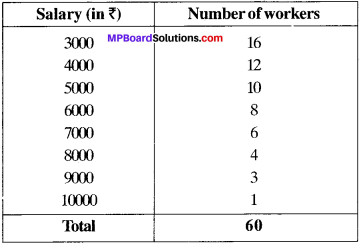Solution: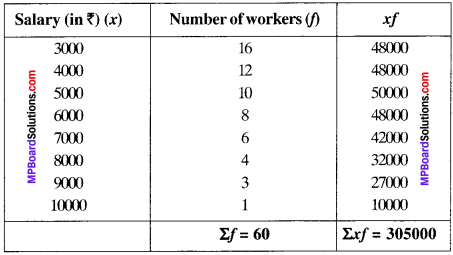Question 6.
Give one example of a situation in which

1. The mean is an appropriate measure of central tendency.
2. The mean is not an appropriate measure of central tendency but the median is an appropriate measure of central tendency.

Solution:
1. Mean is a quantitative central tendency of a data.
Example:
For measuring central tendency of marks of a test we find the mean of the data.

2. Median is a qualitative central tendency of a data.
Example:
For measuring central tendency of beauty of a group of women, we determine the median of the data.